# Move the Matches Puzzles Episode 12 Levels 41-60 Walkthrough

Walkthrough for all the solutions and answers to the game Move the Matches Puzzles by K-Lab. Get the app on the Google Play Store to play along if you do not already have it. This is for episode 12, levels 41 through 60.

For the walkthrough (or answer) images, we will be showing the final image and answer of the Matches Puzzle. This will allow you to see where everything goes, and to know for sure that the puzzle works.  These puzzles have been recently updated, so other walkthroughs do not have the updated puzzles, but we try to always stay up to date with every walkthrough and answer!

### Level 41Instructions: Move 2 matches to correct the equation

Solution: 11-5=6

### Level 42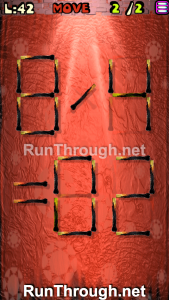Instructions: Move 2 matches to correct the equation

Solution: 8/4=02

### Level 43Instructions: Move 3 matches to correct the equation

Solution: 8×6=48

### Level 44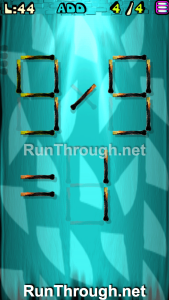Instructions: Add 4 matches to correct the equation

Solution: 9/9=1

### Level 45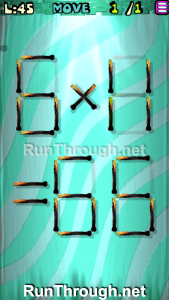Instructions: Move 1 match to correct the equation

Solution: 6×11=66

### Level 46Instructions: Move 2 matches to correct the equation

Solution: 3×3=09

### Level 47Instructions: Move 3 matches to correct the equation

Solution: 7+6=13

### Level 48Instructions: Add 4 matches to correct the equation

Solution: 8-1=07

### Level 49Instructions: Move 2 matches to correct the equation

Solution: 8-8=00

### Level 50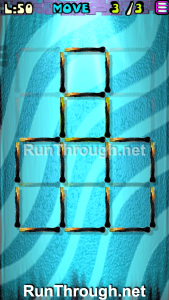Instructions: Move 3 matches to get 4 squares

### Level 51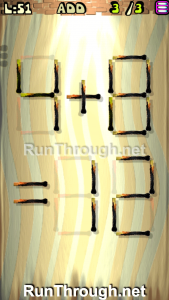Instructions: Add 3 matches to correct the equation

Solution: 4+8=12

### Level 52Instructions: Remove 4 matches to correct the equation

Solution: 5+1=06

### Level 53Instructions: Move 2 matches to correct the equation

Solution: 5-0=05

### Level 54Instructions: Move 1 match to correct the equation

Solution: 2+3=05

### Level 55Instructions: Move 2 matches to correct the equation

Solution: 11-0=011

### Level 56Instructions: Move 2 matches to correct the equation

Solution: 9×3=27

### Level 57Instructions: Move 3 matches to correct the equation

Solution: 3+8=011

### Level 58Instructions: Move 1 match to correct the equation

Solution: 8-9=-1

### Level 59Instructions: Add 3 matches to correct the equation

Solution: 9×7=63

### Level 60Instructions: Move 3 matches to get 4 squares